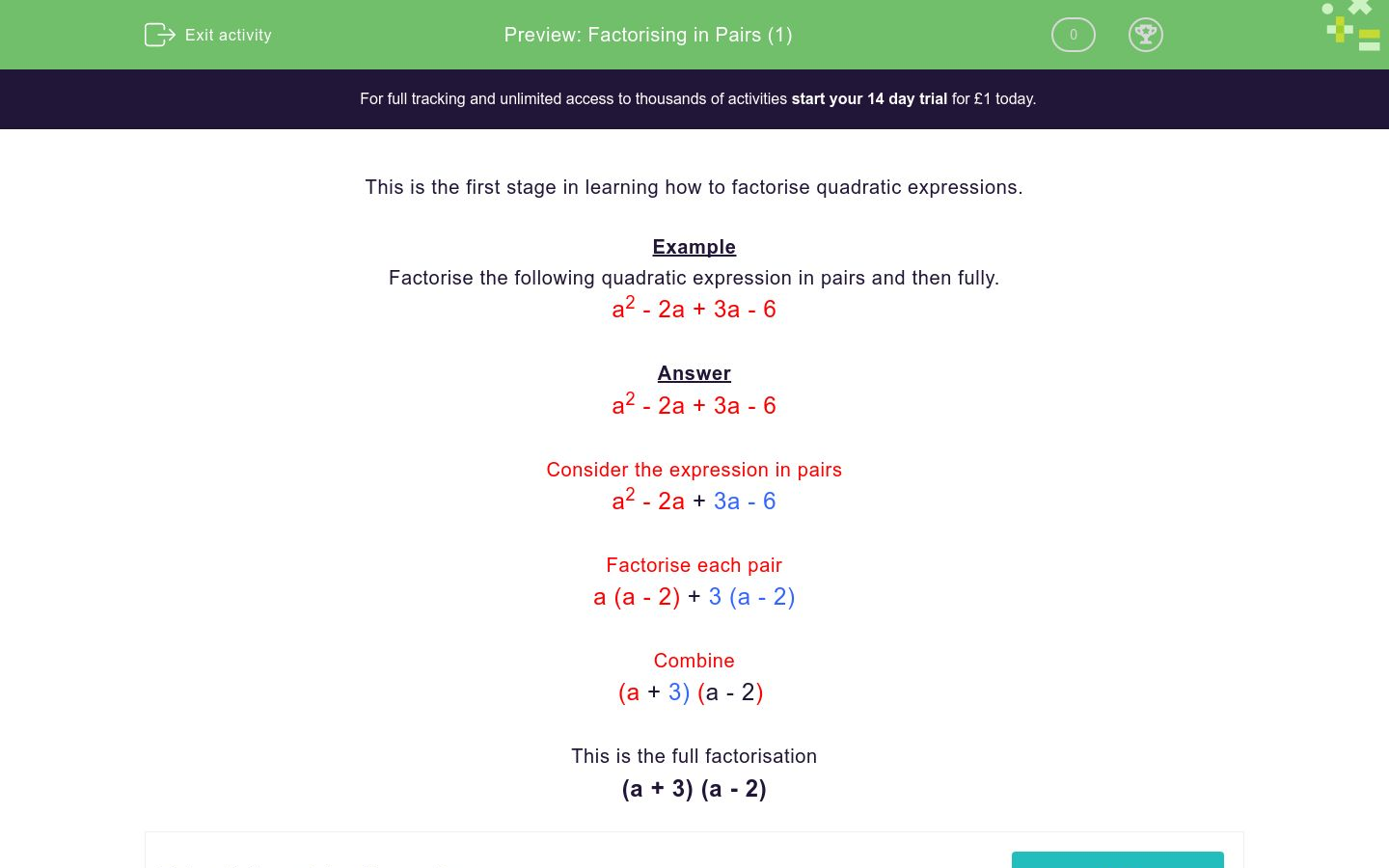# Factorising in Pairs (1)

In this worksheet, students factorise quadratic expressions in pairs.Key stage:  KS 4

Curriculum topic:  Algebra

Curriculum subtopic:  Simplify/Manipulate Algebraic Expressions/Fractions

Difficulty level:### QUESTION 1 of 10

This is the first stage in learning how to factorise quadratic expressions.

Example

Factorise the following quadratic expression in pairs and then fully.

a2 - 2a + 3a - 6

a2 - 2a + 3a - 6

Consider the expression in pairs

a2 - 2a + 3a - 6

Factorise each pair
a (a - 2) + 3 (a - 2)

Combine

(a + 3) (a - 2)

This is the full factorisation

(a + 3) (a - 2)

Factorise the following quadratic expression in pairs and then fully.

a2 + 2a + 3a + 6

(a + 2) (a + 6)

(a + 1) (a + 6)

(a + 3) (a + 2)

(a + 2) (a + 4)

Factorise the following quadratic expression in pairs and then fully.

a2 + 6a + 2a + 12

(a + 2) (a + 6)

(a + 1) (a + 6)

(a + 3) (a + 2)

(a + 2) (a + 4)

Factorise the following quadratic expression in pairs and then fully.

a2 - 4a + 2a - 8

(a - 2) (a + 6)

(a + 1) (a - 6)

(a - 3) (a - 2)

(a + 2) (a - 4)

Factorise the following quadratic expression in pairs and then fully.

a2 - 6a + a - 6

(a - 2) (a + 6)

(a + 1) (a - 6)

(a - 3) (a - 2)

(a + 2) (a - 4)

Factorise the following quadratic expression in pairs and then fully.

a2 + 6a - 2a - 12

(a - 2) (a + 6)

(a + 1) (a - 6)

(a - 3) (a - 2)

(a + 2) (a - 4)

Factorise the following quadratic expression in pairs and then fully.

a2 + 3a - 2a - 6

(a - 2) (a + 3)

(a + 2) (a - 6)

(a - 3) (a - 2)

(a + 3) (a - 4)

Factorise the following quadratic expression in pairs and then fully.

a2 - 2a - 3a + 6

(a - 2) (a + 3)

(a + 2) (a - 6)

(a - 3) (a - 2)

(a + 3) (a - 4)

Factorise the following quadratic expression in pairs and then fully.

a2 - 4a + 3a - 12

(a - 3) (a + 4)

(a + 2) (a - 6)

(a - 6) (a - 2)

(a + 3) (a - 4)

Factorise the following quadratic expression in pairs and then fully.

a2 + 4a - 3a - 12

(a - 3) (a + 4)

(a + 2) (a - 6)

(a - 6) (a - 2)

(a + 3) (a - 4)

Factorise the following quadratic expression in pairs and then fully.

a2 - 9a + 2a - 18

(a - 2) (a + 9)

(a + 2) (a - 9)

(a - 6) (a - 3)

(a + 3) (a - 6)

• Question 1

Factorise the following quadratic expression in pairs and then fully.

a2 + 2a + 3a + 6

(a + 3) (a + 2)
• Question 2

Factorise the following quadratic expression in pairs and then fully.

a2 + 6a + 2a + 12

(a + 2) (a + 6)
• Question 3

Factorise the following quadratic expression in pairs and then fully.

a2 - 4a + 2a - 8

(a + 2) (a - 4)
• Question 4

Factorise the following quadratic expression in pairs and then fully.

a2 - 6a + a - 6

(a + 1) (a - 6)
• Question 5

Factorise the following quadratic expression in pairs and then fully.

a2 + 6a - 2a - 12

(a - 2) (a + 6)
• Question 6

Factorise the following quadratic expression in pairs and then fully.

a2 + 3a - 2a - 6

(a - 2) (a + 3)
• Question 7

Factorise the following quadratic expression in pairs and then fully.

a2 - 2a - 3a + 6

(a - 3) (a - 2)
• Question 8

Factorise the following quadratic expression in pairs and then fully.

a2 - 4a + 3a - 12

(a + 3) (a - 4)
• Question 9

Factorise the following quadratic expression in pairs and then fully.

a2 + 4a - 3a - 12

(a - 3) (a + 4)
• Question 10

Factorise the following quadratic expression in pairs and then fully.

a2 - 9a + 2a - 18